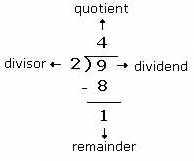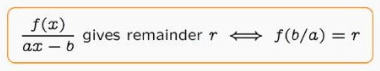# Finding out Remainders Notes | Study Quantitative Aptitude for GMAT - GMAT

## GMAT: Finding out Remainders Notes | Study Quantitative Aptitude for GMAT - GMAT

The document Finding out Remainders Notes | Study Quantitative Aptitude for GMAT - GMAT is a part of the GMAT Course Quantitative Aptitude for GMAT.
All you need of GMAT at this link: GMAT

Remainders is a very crucial concept since numerous questions from Quantitative Aptitude section require the concepts of remainder to solve them. Most of the candidates have already studied this concept in their elementary schools and can solve the related questions. Here is a lesson on Remainders to help the candidates revise the topic in an efficient way.

What is Remainder?
Supposing a number “N” is divided by another number “x”; if the quotient obtained is “Q” and the remainder obtained is “R”, then the number can be expressed as N = Qx + R.

Example: Suppose 9 is divided by 2.
In this case, N = 9, x = 2,  2 × 4 = 8, which is 1 less than 9. hence Q = 4 and R = (9-8) = 1 . Hence, 9 = 4 × 2 + 1.Basic Remainder Theorem
The basic remainder theorem is based on the product of individual remainders.If R is the remainder of an expression( p*q*r)/X, and pR, qR and rR are the remainders when p,q and r are respectively divided by X, then it can be said that ((pR × qR × rR ))/X, will give the same remainder as given by (p*q*r)/X.
Let’s understand this with the help of some examples.

1. Find the remainder when (361*363) is divided by 12.

Steps:

• Take the product of individual remainders, i.e. 361/12|R =1 and 363/12|R = 3
• Find the remainder when you divide that product by the number (361 × 363)/12|R= (1 × 3)/12|R. answer= 3
This is Basic Remainder theorem put across in Numbers.

2. Find the remainder when 106 is divided by 7 i.e. (106/7)R.
Solution.
106=10x 103
Thus (106/7)R = (103/7 × 103/7)R = ((6 * 6)/7)R = (36/7)R = 1.
So the remainder is 1.
“Remainder when the product of some numbers is divided by the requisite number is the product of individual remainders of the numbers”– This is Basic Remainder Theorem put across in words.

Concept of Negative Remainder
The remainder obtained by division of a number N by a divisor X can be expressed in two ways as “R” and “X - R”
Example: 10/11 remainder is +10 itself. It can also be written as 10-11= -1 Similarly, 32/10 remainder is +2 or -8
Let’s express the solution for questions 31 above, in another way- based on the concept of negative remainder.
Thus (106/7)R = (103/7 × 103/7)R = ((-1 * -1)/7)R = (1/7)R = 1.

Let’s see why this happens:
If the numbers N1, N2, N3 give remainders of R1, R2, R3 with quotients Q1, Q2, Q3 when divided by a common divisor D.

N1 = DQ1 + R1 N2 = DQ2 + R2 N3 = DQ3 + R3

Multiplying = N× N2 × N3

= (DQ+ R1) × (DQ2 + R2) × (DQ3 + R3)
= D(some number) + (R1 × R2 × R3) = first part is divisible by D,
Hence you need to check for the individual remainders only.

The document Finding out Remainders Notes | Study Quantitative Aptitude for GMAT - GMAT is a part of the GMAT Course Quantitative Aptitude for GMAT.
All you need of GMAT at this link: GMATUse Code STAYHOME200 and get INR 200 additional OFF

## Quantitative Aptitude for GMAT

75 videos|87 docs|156 tests

Track your progress, build streaks, highlight & save important lessons and more!

,

,

,

,

,

,

,

,

,

,

,

,

,

,

,

,

,

,

,

,

,

;